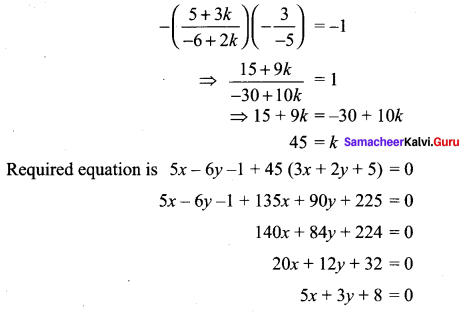## Tamilnadu Samacheer Kalvi 11th Maths Solutions Chapter 6 Two Dimensional Analytical Geometry Ex 6.3

Question 1.
Show that the lines are 3x + 2y + 9 = 0 and 12x + 8y – 15 = 0 are parallel lines.
Solution: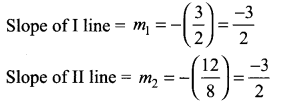Here m1 = m2 ⇒ the two lines are parallel.

Question 2.
Find the equation of the straight line parallel to 5x – 4y + 3 = 0 and having x – intercept 3.
Solution:
Equation of a line parallel to ax + by + c = 0 will be of the form ax + by + k = 0
So equation of a line parallel to 5x – 4y + 3 = 0 will be of the form 5x – 4y = k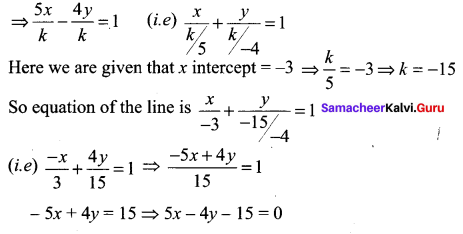Question 3.
Find the distance between the line 4x + 3y + 4 = 0 and a point
(i) (-2, 4)
(ii) (7, -3)
Solution:
The distance between the line ax + by + c = 0 and the point(x1, y1) is given by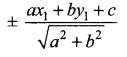(i) Now the distance between the line 4x + 3y + 4 = 0 and (-2, 4) is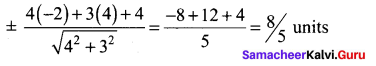(ii) The distance between the line 4x + 3y + 4 = 0 and (7, -3) is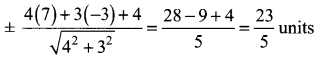Question 4.
Write the equation of the lines through the point (1, -1)
(i) Parallel to x + 3y – 4 = 0
(ii) Perpendicular to 3x + 4y = 6
Solution:
(i) Any line parallel to x + 3y – 4 = 0 will be of the form x + 3y + k = 0.
It passes through (1,-1) ⇒ 1 – 3 + k = 0 ⇒k = 2
So the required line is x + 3y + 2 = 0

(ii) Any line perpendicular to 3x + 4y – 6 = 0 will be of the form 4x – 3y + k = 0.
It passes through (1, -1) ⇒ 4 + 3 + k = 0 ⇒ k = -7.
So the required line is 4x – 3y – 7 = 0

Question 5.
If (- 4, 7) is one vertex of a rhombus and if the equation of one diagonal is 5x – y + 7 = 0, then find the equation of another diagonal.
Solution:
In a rhombus, the diagonal cut at right angles.
The given diagonal is 5x – y + 7 = 0 and (- 4, 7) is not a point on the diagonal.
So it will be a point on the other diagonal which is perpendicular to 5x – y + 7 = 0.
The equation of a line perpendicular to 5x – y + 7 = 0 will be of the form x + 5y + k = 0. It passes through (-4, 7) ⇒ -4 + 5(7) + k = 0 ⇒ k = -31
So the equation of the other diagonal is x + 5y – 31 = 0

Question 6.
Find the equation of the lines passing through the point of intersection lines 4x – y + 3 = 0 and 5x + 2y +7 = 0, and
(i) Through the point (-1, 2)
(ii) Parallel to x – y + 5 = 0
(iii) Perpendicular to x – 2y + 1 = 0.
Solution:
To find the point of intersection of the lines we have to solve them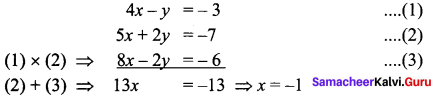Substituting x = -1 in equation (2) we get
-5 + 2y = -7
⇒ 2y = – 7 + 5 = -2
⇒ y = -1
So the point of intersection is (-1, -1)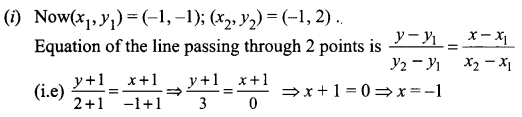(ii) Equation of a line parallel to x – y + 5 = 0 will be of the form x – y + k= 0.
It passes through (-1, -1) ⇒ -1 + 1 + k = 0 ⇒ k = 0.
So the required line is x – y = 0 ⇒ x = y.

(iii) Equation of a line perpendicular to x – 2y+ 1 =0 will be of the form 2x + y + k = 0. It passes through (-1, -1) ⇒ -2 – 1 + k = 0 ⇒ k = 3.
So the required line is 2x + y + 3 = 0Question 7.
Find the equations of two straight lines which are parallel to the line 12x + 5y + 2 = 0 and at a unit distance from the point (1, -1).
Solution:
Equation of a line parallel to 12x + 5y + 2 = 0 will be of the form 12x + 5y + k = 0.
We are given that the perpendicular distance form (1, -1) to the line 12x + 5y + k = 0 is 1 unit.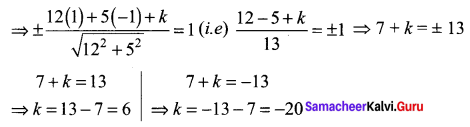So the required line will be 12x + 5y + 6 = 0 or 12x + 5y – 20 = 0

Question 8.
Find the equations of straight lines which are perpendicular to the line 3x + 4y – 6 = 0 and are at a distance of 4 units from (2, 1).
Solution:
Given equation of line is 3x + 4y – 6 = 0.
Any line perpendicular to 3x + 4y – 6 = 0 will be of the form 4x – 3y + k = 0
Given perpendicular distance is 4 units from (2, 1) to line (1)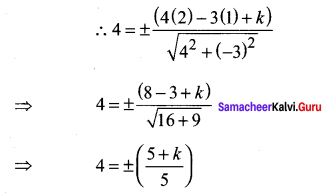∴ 20 = + (5 + k) or 20 = – (5 + k)
⇒ k = 20 – 5 or k = -(20 + 5)
k = 15 or k : = -25
∴ Required equation of the lines are 4x – 3y + 15 = 0 and 4x – 3y – 25 = 0

Question 9.
Find the equation of a straight line parallel to 2x + 3y = 10 and which is such that the sum of its intercepts on the axes is 15.
Solution:
The equation of the line parallel to 2x + 3y = 10 will be of the form 2x + 3y = k .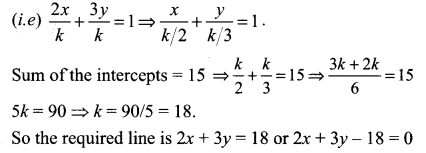Question 10.
Find the length of the perpendicular and the co-ordinates of the foot of the perpendicular from (-10, -2) to the line x + y – 2 = 0.
Solution:
Length of the perpendicular from (-10, -2) to x + y – 2 = 0 is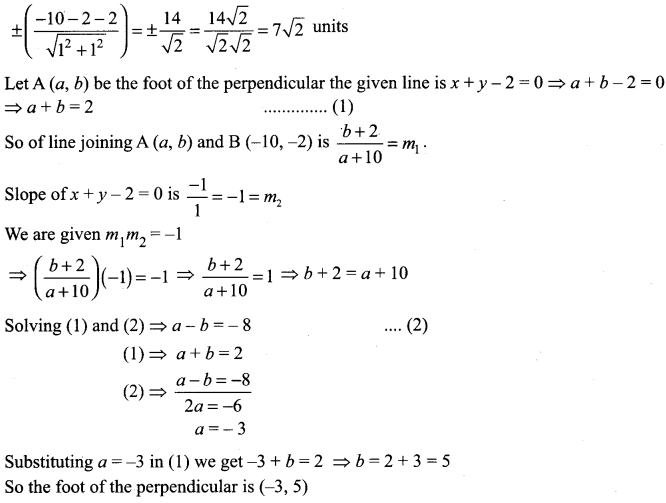Question 11.
If p1 and p2 are the lengths of the perpendiculars from the origin to the straight lines. sec θ +y cosec θ = 2a and x cos θ – y sin θ = a cos 2θ, then prove that p_{1}^{2}+p_{2}^{2}=a^{2}
Solution:
p1 = length of perpendicular from (0, 0) to x sec θ + y cosec θ = 2a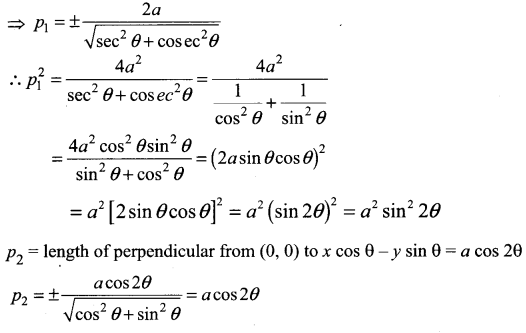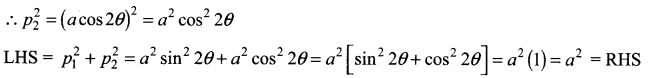Question 12.
Find the distance between the parallel lines
(i) 12x + 5y = 7 and 12x + 5y + 7 = 0
(ii) 3x – 4y + 5 = 0 and 6x – 8y – 15 = 0.
Solution: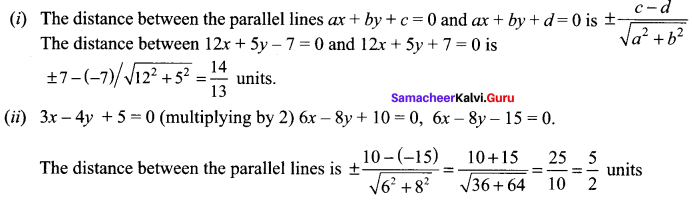Question 13.
Find the family of straight lines
(i) Perpendicular
(ii) Parallel to 3x + 4y – 12 = 0.
Solution:
Equation of lines perpendicular to 3x + 4y – 12 = 0 will be of the form 4x – 3y + k = 0, k ∈ R Equation of lines parallel to 3x + 4y – 12 = 0 will be of the form 3x + 4y + k = 0, k ∈ R

Question 14.
If the line joining two points A (2, 0) and B (3, 1) is rotated about A in anti-clockwise direction through an angle of 15°, then find the equation of the line in new position.
Solution: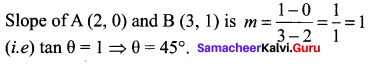This line is rotated about 15° in anti clockwise direction
⇒ New slope = tan (45° + 15°) = tan 60° = $$\sqrt{3}$$ (i.e) m = $$\sqrt{3}$$.
Point A = (2, 0)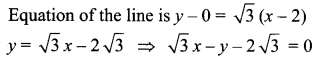Question 15.
A ray of light coming from the point (1, 2) is reflected at a point A on the x-axis and it passes through the point (5,3). Find the co-ordinates of the point A.
Solution:
The image of the point P (1, 2) will be P’ (1, -2).
Since ∠OAP = ∠XAQ (angle of inches = angle of reflection) So ∠OAP’ = ∠XAQ = a (Vertically opposite angles)
⇒ P’, A, Q lie on a same line.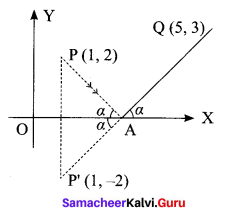Now equation of the line P’, Q is [where P’ = (1, -2), Q = (5, 3)]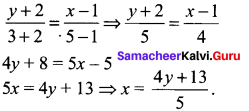Since we find point of intersection with x axis we put y = 0.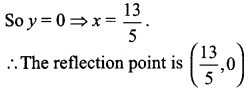Question 16.
A line is drawn perpendicular to 5x = y + 7. Find the equation of the line if the area of the triangle formed by this line with co-ordinate axes is 10 sq. units.
Solution:
Equation of the given lines 5x = y+ 7 ⇒ 5x – y = 7.
So its perpendicular will be of the form x + 5y = 7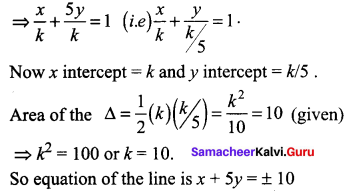Question 17.
Find the image of the point (-2, 3) about the line x + 2y – 9 = 0.
Solution:
The coordinates of image of the point (x1, y1) with respect to the line ax + by + c = 0 can be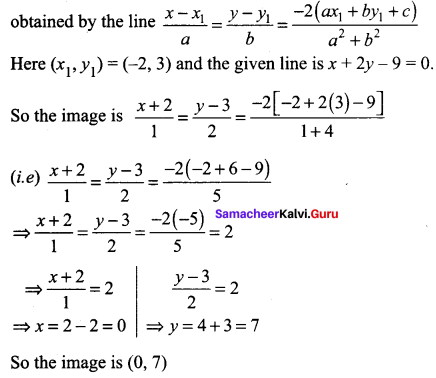Question 18.
A photocopy store charges Rs. 1.50 per copy for the first 10 copies and Rs. 1.00 per copy after the 10th copy. Let x be the number of copies, and let y be the total cost of photocopying.
(i) Draw graph of the cost as x goes from 0 to 50 copies.
(ii) Find the cost of making 40 copies
Solution: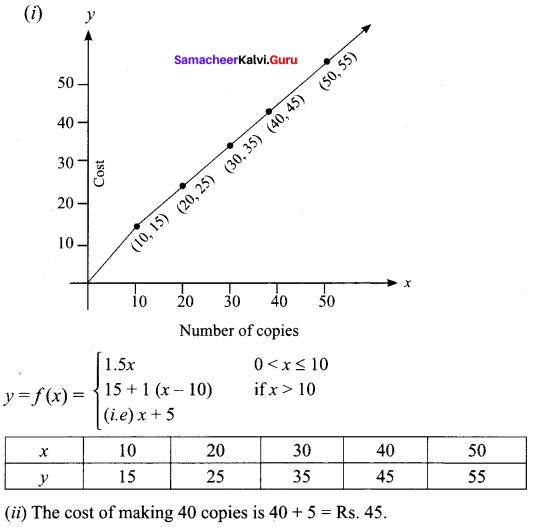Question 19.
Find atleast two equations of the straight lines in the family of the lines y = 5x + b, for which b and the x-coordinate of the point of intersection of the lines with 3x – 4y = 6 are integers.
Solution:
y = 5x + b …….. (1)
3x-4y = 6 …….. (2)
Solving (1) and (2)
Substituting y value from (1) in (2) we get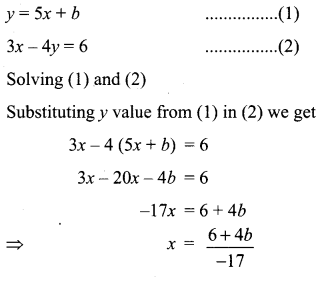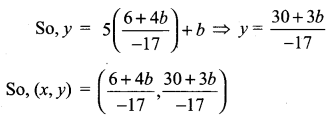Since, x coordinate and 6 are integers 6 + 46 must be multiple of 17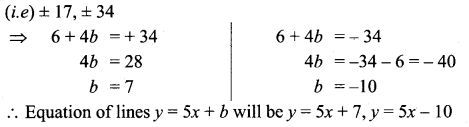Question 20.
Find all the equations of the straight lines in the family of the lines y = mx – 3, for which m and the x-coordinate of the point of intersection of the lines with x – y = 6 are integers.
Solution:
Equation of the given lines are
y= mx – 3 …….. (1)
and x – y = 6 ……. (2)
Solving (1) and (2)
x – (mx – 3) = 6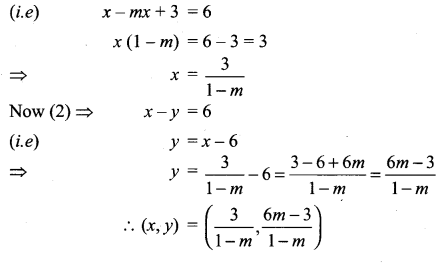Since m and x coordinates are integers
1 – m is the divisor of 3 (i.e) ± 1, ± 3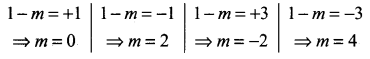So equation of lines are (y = mx – 3) ,y = mx – 3
(i) When m = 0, y = -3
(ii) When m = 2, y = 2x – 3
(iii) When m = -2, y = -2x – 3 or 2x + y + 3 = 0
(iv) When m = 4, y = 4x – 3

### Samacheer Kalvi 11th Maths Solutions Chapter 6 Two Dimensional Analytical Geometry Ex 6.3 Additional Questions

Question 1.
Find the equation of the line passing through the point (5, 2) and perpendicular to the line joining the points (2, 3) and (3, -1).
Solution:
Slope of the line joining the points (2, 3) and (3, -1) is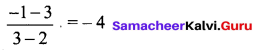Slope of the required line which is perpendicular to it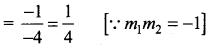Equation of the line passing through the point (5, 2) is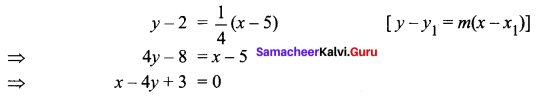Hence, the required equation is x – 4y + 3 = 0.

Question 2.
Find the points on the line x + y = 4 which lie at a unit distance from the line 4x + 3y = 10. Solution: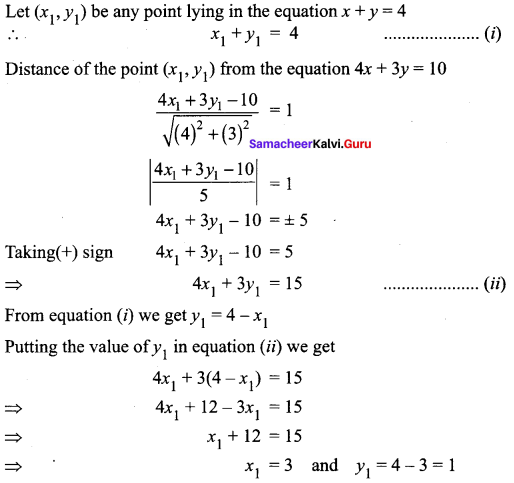So, the required point is (3, 1)
Now taking(-) sign, we have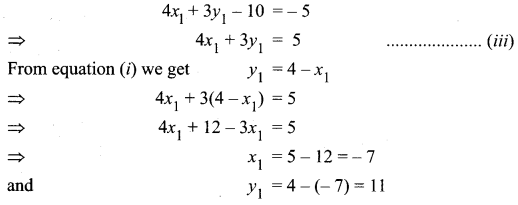So, the required point is (- 7, 11)
Hence, the required points on the given line are (3, 1) and (-7, 11).Question 3.
Find the equation of the line passing through the point of intersection 2x + y = 5 and x + 3y + 8 = 0 and parallel to the line 3x +4y = 7.
Solution: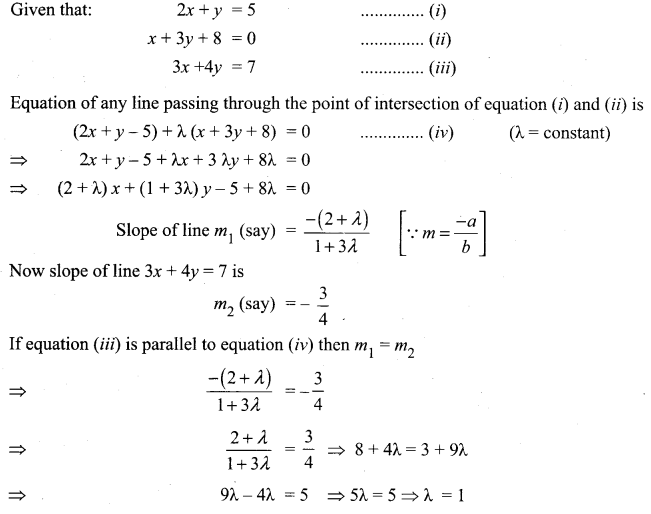On putting the value of λ in equation (iv) we get
(2x + y – 5) + 1 (x + 3y + 8) = 0
⇒ 2x + y – 5 + x + 3y + 8 = 0
⇒ 3x + 4y + 3 = 0
Hence, the required equation is 3x + 4y + 3 = 0

Question 4.
A line passing through the points (a, 2a) and (-2, 3) is perpendicular to the line 4 x + 3y + 5 = 0, find the value of a.
Solution: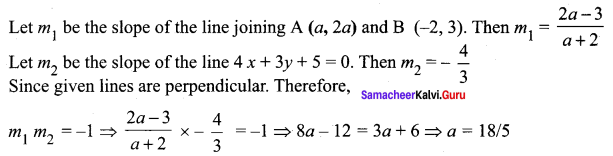Question 5.
Find the equation of the straight line which passes through the intersection of the straight lines 2x + y = 8 and 3x – 2y + 7 = 0 and is parallel to the straight line 4x + y – 11 = 0.
Solution:
Equation of line through the intersection of straight lines
2x + y = 8 and 3x – 2y + 7 = 0 is
2x + y – 8 + k (3x – 2y + 7) = 0
x(2 + 3k) + y (1 – 2k) +(-8 + 7k) = 0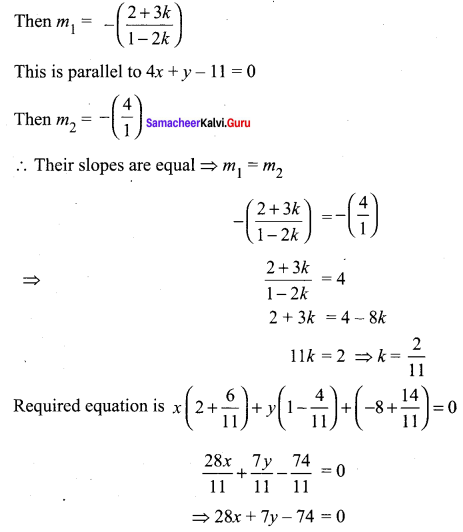⇒ 28x + 7y – 74 = 0

Question 6.
Find the equation of the straight line passing through intersection of the straight lines 5x – 6y = 1 and 3x + 2y + 5 = 0 and perpendicular to the straight line 3x – 5y + 11 = 0.
Solution:
Equation of line through the intersection of straight lines 5x – 6y = 1 and 3x + 2y + 5 = 0 is
5x – 6y – 1 + k (3x + 2y + 5) = 0
x (5 + 3k) + y (-6 + 2k) + (-1 + 5k) = 0
This is perpendicular to 3x – 5y + 11 = 0
That is, the product of their slopes is -1